Geometry

# Length and Area: Level 3 Challenges

Triangles $ABC$ and $XYZ$ are congruent right angled isosceles triangles. Squares blue and red are as shown. If the area of blue square is $189$. What is the area of the red square?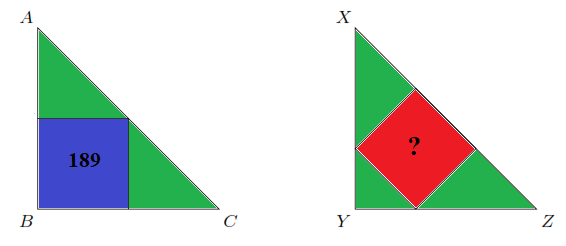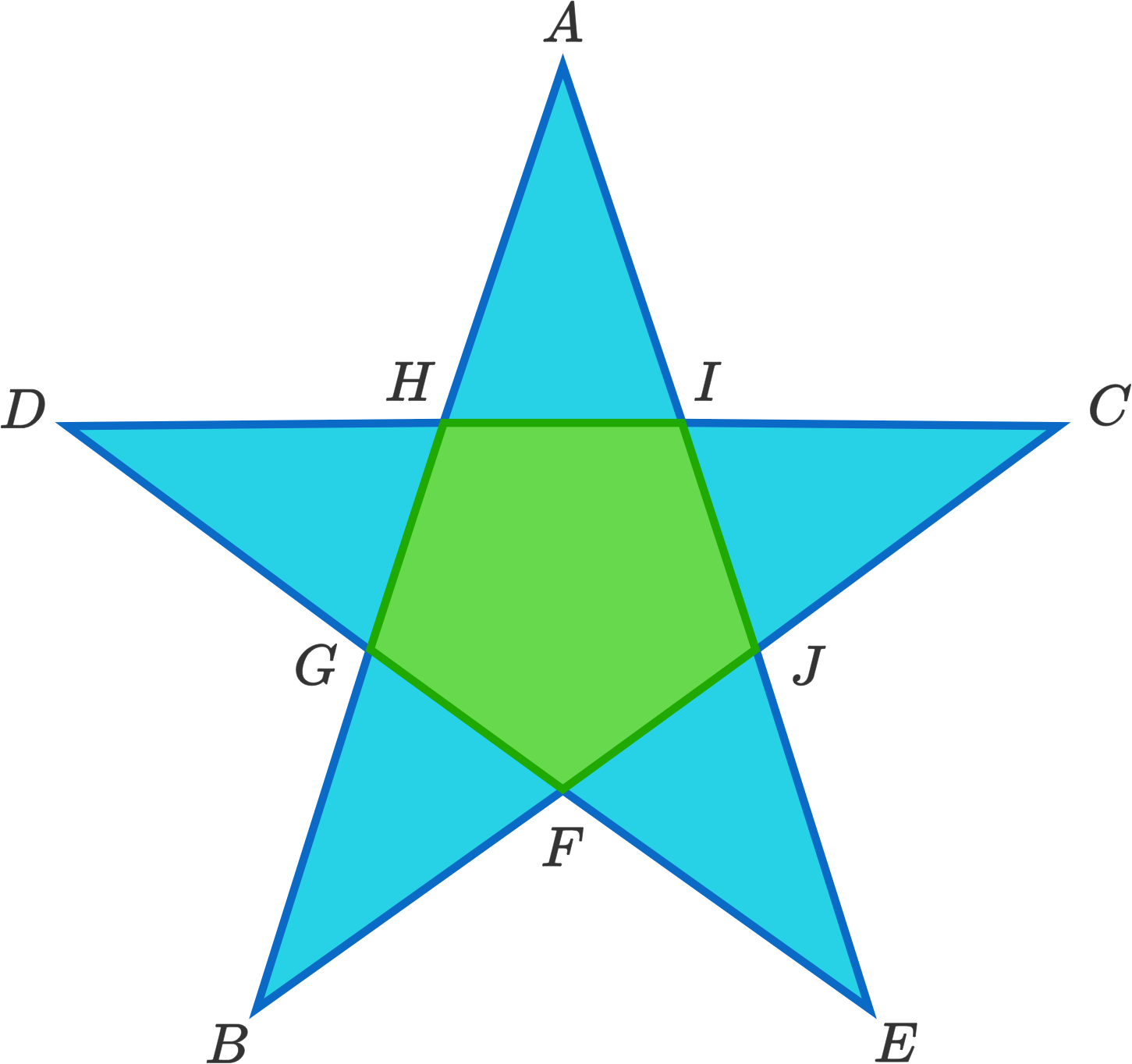If the star has an outside perimeter of 50, and the pentagon has a perimeter of 30, what is $AB + BC + CD + DE + EA$?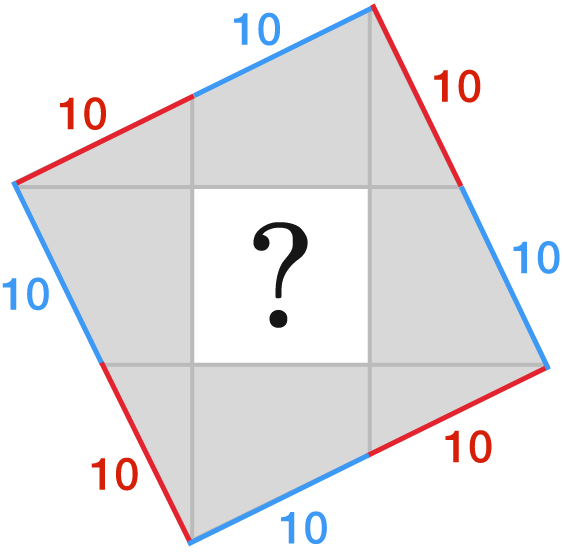Line segments are drawn from the vertices of the large square to the midpoints of the opposite sides to form a smaller, white square.

If each red or blue line-segment measures $10$ m long, what is the area of the smaller, white square in m$^{2}?$Three semicircles (with equal radii) are drawn inside the large semicircle so that their diameters all sit on the diameter of the large semicircle. What is the ratio of the red area to the blue area?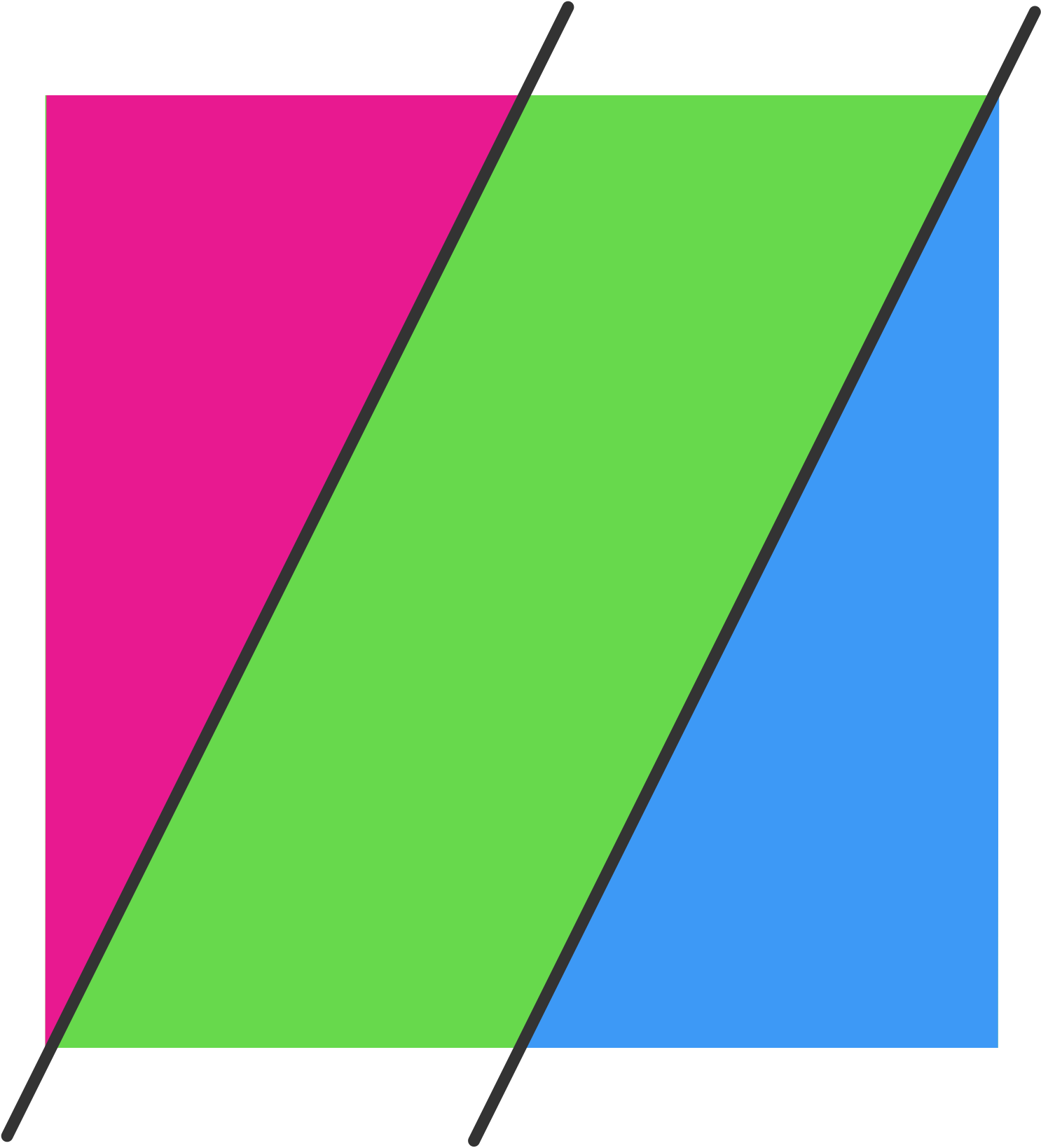Suppose a square is cut by two parallel lines at perpendicular distance $6$ cm. apart such that

(i) one line has an endpoint at the lower left corner of the square and the other has an endpoint at the upper right corner of the square, and

(ii) the square is divided into three regions, (a parallelogram and two triangles), of equal area.

What is the area of the square in cm$^{2}$?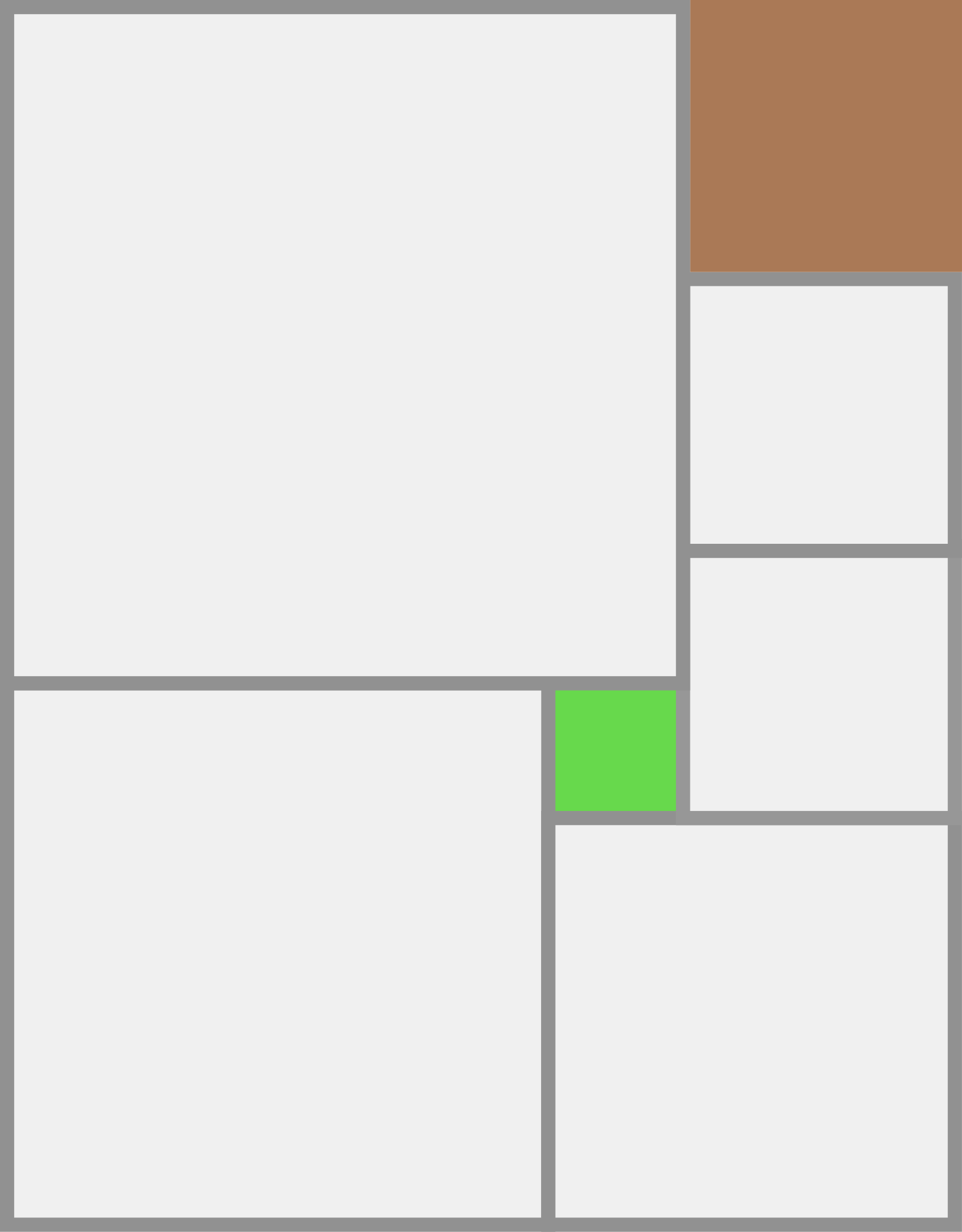There are 7 smaller squares inside the large rectangle above.

If the green square has side length 1 and the brown square has side length 2, what is the area of the rectangle?

×